# THÉVENIN’S THEOREMClick or Tap the Example circuits below to invoke TINACloud and select the Interactive DC mode to Analyze them Online.
Get a low cost access to TINACloud to edit the examples or create your own circuits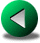POWER IN DC CIRCUITSNORTON’S THEOREM

Thévenin’s Theorem allows one to replace a complicated circuit with a simple equivalent circuit containing only a voltage source and a series connected resistor. The theorem is very important from both theoretical and practical viewpoints.

Concisely stated, Thévenin’s Theorem says:

Any two-terminal linear circuit can be replaced by an equivalent circuit consisting of a voltage source (VTh) and a series resistor (RTh).

It is important to note that the Thévenin equivalent circuit provides equivalence at the terminals only. Obviously, the internal structure and therefore the characteristics of the original circuit and the Thévenin equivalent are quite different.

Using Thevenin's theorem is especially advantageous when:

• We want to concentrate on a specific portion of a circuit. The rest of the circuit can be replaced by a simple Thevenin equivalent.
• We have to study the circuit with different load values at the terminals. Using the Thevenin equivalent we can avoid having to analyze the complex original circuit each time.

We can calculate the Thevenin equivalent in two steps:

1. Calculate RTh. Set all sources to zero (replace voltage sources by short circuits and current sources by open circuits) and then find the total resistance between the two terminals.
2. Calculate VTh. Find the open circuit voltage between the terminals.

To illustrate, let's use Thévenin’s Theorem to find the equivalent circuit of the circuit below.

Click/tap the circuit above to analyze on-line or click this link to Save under Windows

The TINA solution shows the steps needed for the calculation of the Thevenin parameters: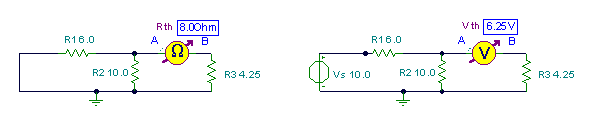Of course, the parameters can be calculated easily using the rules of series-parallel circuits described in previous chapters: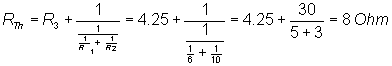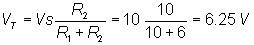Further examples:

Example 1

Here you can see how the Thévenin equivalent simplifies calculations.

Find the current of the load resistor R if its resistance is:

1.) 0 ohm; 2.) 1.8 ohm; 3.) 3.8 ohm 4.) 2.8.ohm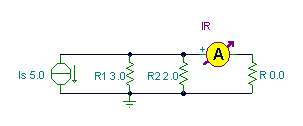First find the Thévenin equivalent of the circuit with respect to the terminals of R, but without R: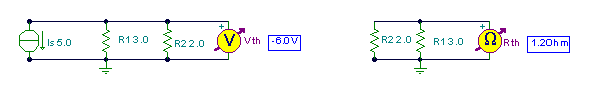Now we have a simple circuit with which it is easy to calculate the current for the different loads:

Click/tap the circuit above to analyze on-line or click this link to Save under Windows

An example with more than one source:

Example 2

Find the Thévenin equivalent of circuit.

Click/tap the circuit above to analyze on-line or click this link to Save under Windows

Solution by TINA's DC analysis: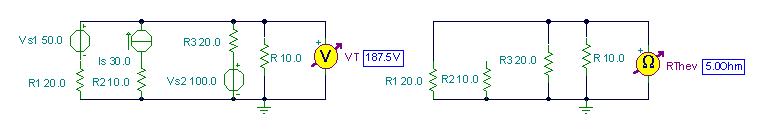The complicated circuit above, then, can be replaced by the simple series circuit below.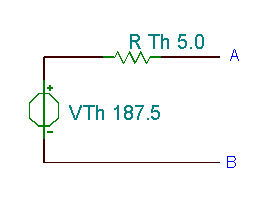POWER IN DC CIRCUITSNORTON’S THEOREM